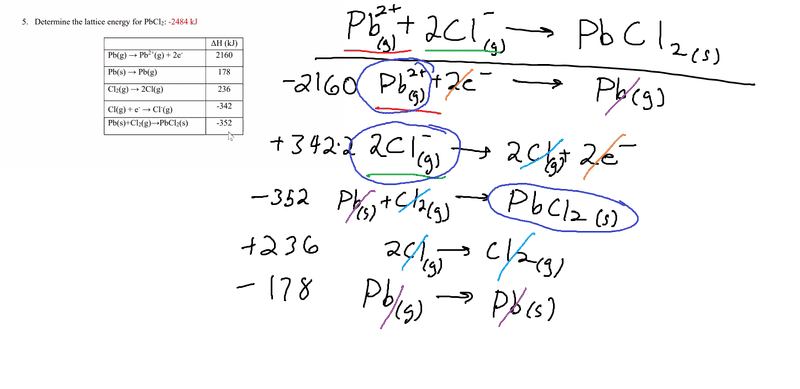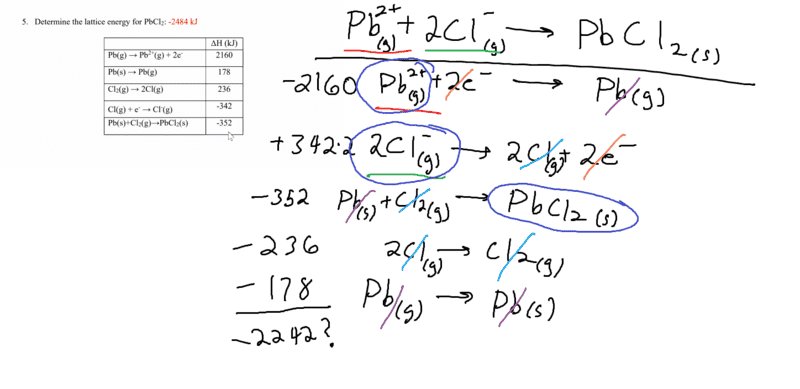# Determine Lattice Energy of PbCl2

• Chemistry
• cuallito

#### cuallito

Homework Statement
Determine the lattice energy of PbCl2
Relevant Equations
Lattice energy= Heat of formation- Heat of atomization- Dissociation energy- (sum of Ionization energies)- (sum of Electron Affinities)
Here's my attempt at solving it using Hess's Law. I get -1770kJ when I add up all the numbers. The correct answer is supposed to be -2484 kJ.I forgot to flip the sign for 2Cl(g)->Cl2(g), but I'm still not getting the right number:I agree with your calculation. Maybe the book's wrong - it happens.

•cuallito